##Ellipse

 The Ellipse can be defined by the property of its points X(x,y): |XF'|+|XF| = 2a, a being a constant and {F,F'} being fixed points, called the focal points of the ellipse. Line FF' is called the major axis. The middle O of segment FF' is the center of the ellipse. Points {A,A'} in which the ellipse intersects the major axis are called vertices of the ellipse. The computations below assume that the major axis coincides with the x-axis of the coordinate system and O coincides with the origin of coordinates.
 a = |AA'|/2 is called also the major axis of the ellipse.
 O is the middle of AA', the circle c centered at O, with radius a is called the auxiliary circle of the ellipse.
 Defining the focal distance, |FF'| = 2c we see easily that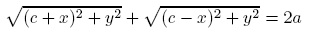Defining b properly, this is equivalent with the equation below, and related to the figure by: |HJ|/|XJ| = a/b, b is called the minor axis.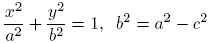Defining the quantities e, h, through the equations of the first line below, the equation of the conic becomes equal to the equation of the second line, depicting another geometric property of the ellipse: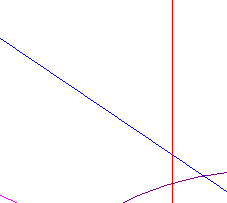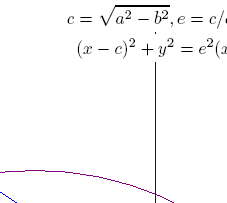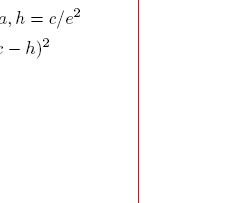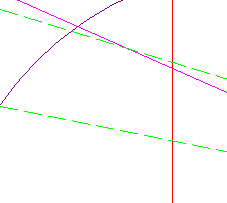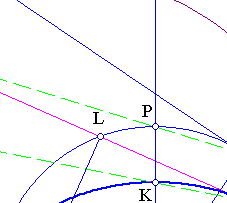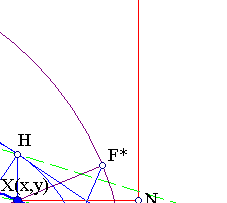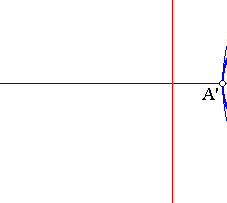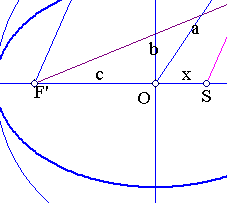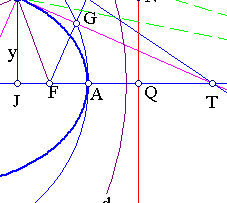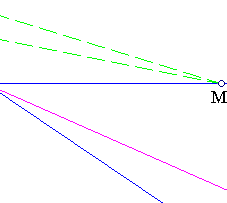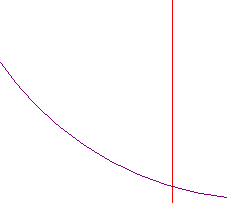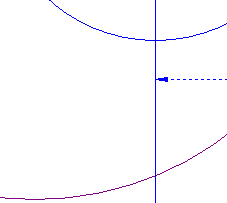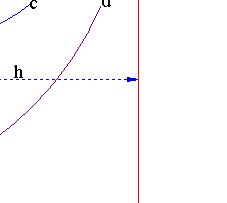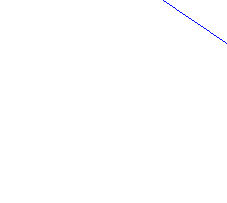Taking the line parallel to the y-axis at Q(h,0), called a directrix, this shows that |XF|/|XN| = e, where e = c/a is called the eccentricity of the ellipse. Thus the ellipse can be identified with the locus of points X, such that the ratio of distances from a point (focus F) and a line (directrix) is constant (=e < 1).
 There is a second directrix: the symmetric of QN w.r. to O, characterized by the same property as the previous one. The two directrices are the polars of the foci, with respect to the conic.
 The direction of the normal at X(x,y) is (x/a2, y/b2), hence the equation of the tangent is xu/a2 + yv/b2 = 1 (variable (u,v)). The points {S,T} where the normal/tangent intersect the x-axis are easily calculated to be at {x(a2-b2)/a2, a2/x}, their product being c2=a2-b2. This implies that {T,S} are harmonic conjugate with respect to {F,F'} and since the angle at X is a right one lines XT, XN coincide with the bisectors of the angle FXF'.
 This implies that the symmetric F* of F with respect to the tangent XT is on XF' and the middle G of FF* is on the auxiliary circle with diameter AA'.
 Circle d, with center at the focus F' and radius 2a is called a major circle of the ellipse. It has the property: For every point F* on d, the medial line of segment FF* is tangent to the ellipse at its intersection point X, with F'F*.
 Projecting a focal point on a tangent, defines a point on the auxiliary circle c (by  OG is parallel and the half length of F'F*).
 Line XJ is simultaneously the polar of T with respect to the ellipse and the auxiliary circle c. Therefore the tangents at X to the ellipse and H to the circle meet at T. Also lines KX and PH meet at the same point on the x-axis since JX/JH = OK/OP = b/a. The file CommonPolar.html contains some consequences of the coincidence of the tangent lines at T.
 All properties of ellipses, discussed so far, have their twins for hyperbolas (see Hyperbola.html ). Ellipses and hyperbolas are the two kinds of conics called collectively central conics, because they have a center of symmetry.